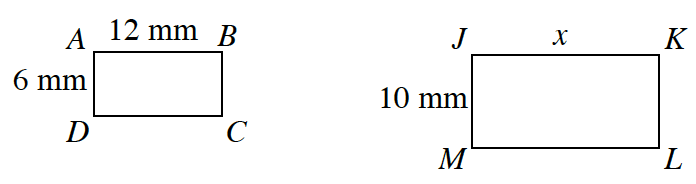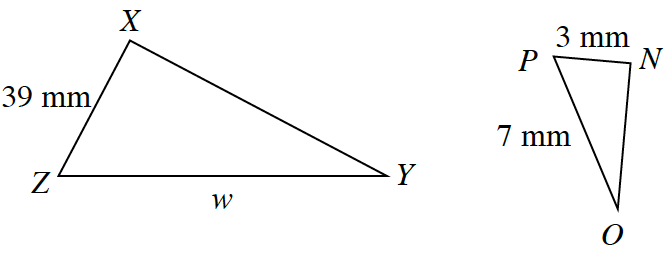Home > GC > Chapter 3 > Lesson 3.2.2 > Problem3-59

3-59.

Solve for the missing lengths in the sets of similar figures below. You may want to set up tables to help you write equations. Homework Help ✎

1. $ABCD\sim JKLM$If each pair of shapes is similar, then

$\frac{6}{12}=\frac{10}{x}$ and $\frac{39}{w}=\frac{3}{7}$.

Start to solve for each variable by multiplying both sides of the equation by the lowest common denominator.

2. $ΔNOP\sim∆XYZ$$w=91$